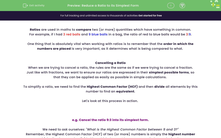# Reduce a Ratio to its Simplest Form

In this worksheet, student will cancel ratios down into their simplest possible forms using the method of converting amounts into the same units then finding the Highest Common Factor.Key stage:  KS 4

Year:  GCSE

GCSE Subjects:   Maths

GCSE Boards:   AQA, Eduqas, Pearson Edexcel, OCR,

Curriculum topic:   Ratio, Proportion and Rates of Change

Curriculum subtopic:   Ratio, Proportion and Rates of Change Calculations with Ratio

Difficulty level:#### Worksheet Overview

Ratios are used in maths to compare two (or more) quantities which have something in common.

For example, if I had 3 red balls and 9 blue balls in a bag, the ratio of red to blue balls would be 3:9.

One thing that is absolutely vital when working with ratios is to remember that the order in which the numbers are placed is very important, as it determines what is being compared to what.

Cancelling a Ratio

When we are trying to cancel a ratio, the rules are the same as if we were trying to cancel a fraction.

Just like with fractions, we want to ensure our ratios are expressed in their simplest possible forms, so that they can be applied as easily as possible in simple calculations.

To simplify a ratio, we need to find the Highest Common Factor (HCF) and then divide all elements by this number to find an equivalent.

Let's look at this process in action.

e.g. Cancel the ratio 9:3 into its simplest form.

We need to ask ourselves: "What is the Highest Common Factor between 9 and 3?"

Remember, the Highest Common Factor (HCF) of two (or more) numbers is simply the highest number which all values can be divided by, which does not leave any remainders or decimals.

The HCF of 9 and 3 is 3, as 3 × 3 = 9 and 3 × 1 = 3.

So we need to divide both sides by 3:

9:3 ÷ 3  -->  3:1

e.g. Simplify the ratio 25:85 as far as possible.

The HCF of 25 and 85 is 5, so we need to divide both sides by 5:

25:85 ÷ 5  -->  5:17

e.g. Write a ratio which compares £2 to 30 p in its simplest possible form.

The key thing to notice here is that the units which our amounts are expressed in <b>are not</b> the same, so we have to convert these into the same units before we start.

There is 100 p in £1, so £2 = 200 p

Remember that the order for our ratio must be the same as in the question:

200 p:30 p

As both our units are now the same, we can remove them:

200:30

Now we can cancel this ratio down by finding the HCF.

The HCF of 200 and 30 is 10, so we need divide both sides by 10:

200:30 ÷ 10  -->  20:3

In this activity, we will cancel ratios down into their simplest possible forms using the method shown above of converting amounts into the same units then finding the Highest Common Factor.

### What is EdPlace?

We're your National Curriculum aligned online education content provider helping each child succeed in English, maths and science from year 1 to GCSE. With an EdPlace account you’ll be able to track and measure progress, helping each child achieve their best. We build confidence and attainment by personalising each child’s learning at a level that suits them.

Get started